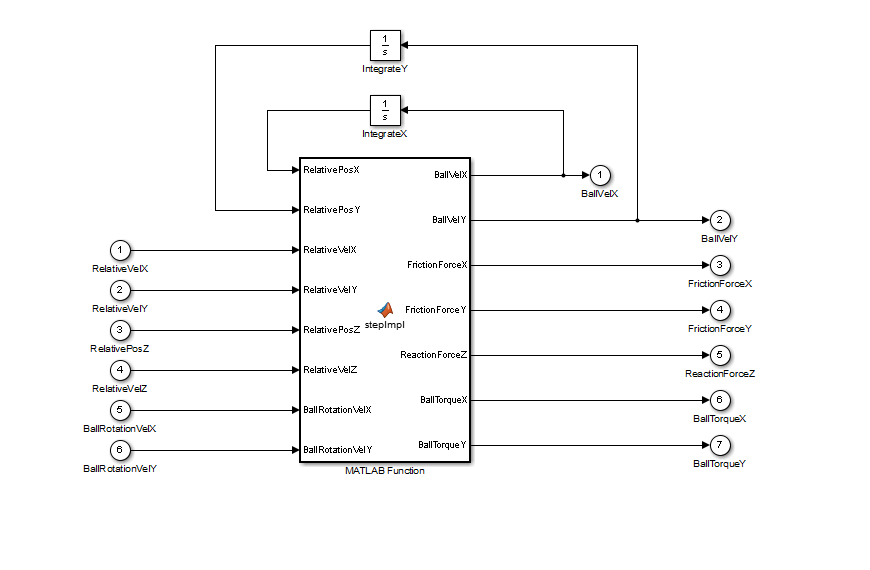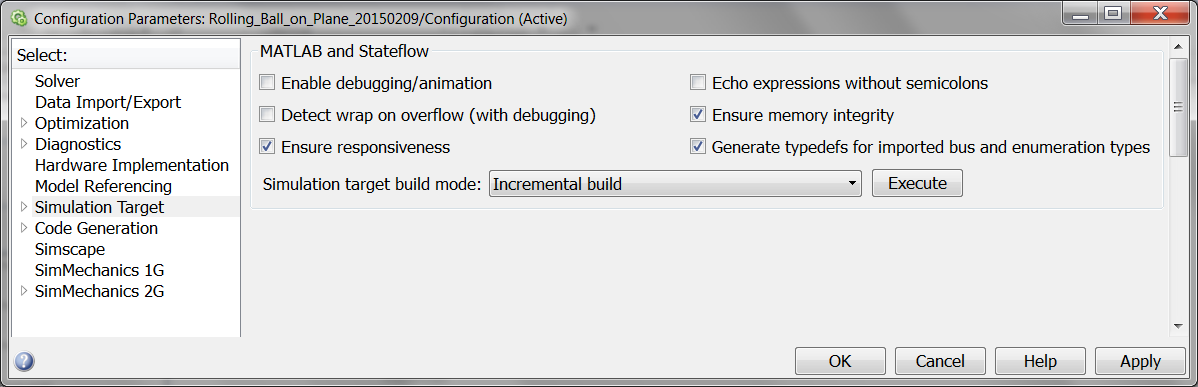# What’s the reaction when we touch?

Contact Modeling in SimMechanics

Greg's pick this week is Rolling Ball on Plane by Janne Salomäki.
Janne put together a nice example of modeling the interaction of a ball contacting a plane, rolling on the plane, and then falling off the plane.

Janne uses SimMechanics to model the geometry and motion of the ball and the plane. And then applies C-code S-Function to model the interaction between the ball and the plane. Contact modeling isn’t something SimMechanics can do, so I’m excited to see an example that shows the basic concept. I also saw an opportunity to compare using C-code and MATLAB Code to solve this particular problem.

### What is SimMechanics?

SimMechanics enables six degree of freedom (6DOF) modeling of rigid bodies in Simulink. A nice benefit is it comes with an animation feature where you can visualize the motion of the bodies. Bodies are defined in terms of mass, inertia tensor, and location for the center of mass. Connections to other bodies are defined by coordinate systems applied to the body, and placing constraints on the type of motion permitted between two coordinate systems. For example, a revolute joint only has one degree of freedom, while a gimbal joint has three degrees of freedom.In this particular case, the joint between the ball and the plane has six degrees of freedom, which means there is no restriction on the relative motion between these two bodies. Using this joint enables measurement and actuation of the relative motions between the two bodies, which is essential for modeling the contact interaction.

### What is an S-function?

An S-Function is a “system function”. It is a way to create customized blocks in Simulink that can extend its functionality. People use S-Functions to for all sorts of capabilities:
• Compile an optimized block algorithm to improve performance
• Protect intellectual property
• Integrate existing code into a Simulink model
While S-functions can be a little tricky, especially if you are unfamiliar with the API, they interact more or less directly with the Simulink solver engine, which enables development of a wide variety of block types and capabilities. Janne uses the C-code S-function to implement the mathematical model for the interaction between the ball and the plane.

### Are there other ways to do this?

There are other ways to develop the interaction model. You could of course use basic Simulink blocks, and develop the equations using Add blocks, Gain blocks, etc. Another option is to write the interaction as MATLAB-Code in the MATLAB Function block. This would enable you to test the algorithm in the flexible MATLAB environment. The MATLAB Function block is not designed to support mathematical integration. So it is often best to pass out the derivatives of the states as outputs, route them through an integrator block, and pass the result back as inputs to the MATLAB Function block.### How do they compare?

C-code S-Function

MATLAB Function

MATLAB Function

(Debugging Disabled)

2.15 sec

2.67 sec

2.30 sec

~

+24%

+6.8%

I thought I would try some basic benchmarking to compare some different implementations. It’s interesting to me that the MATLAB Function block is only about 7% slower. Remember that by default, the MATLAB Function block converts the MATLAB Code to C-code and then compiles it to essentially an S-Function.

MATLAB Debugging DisabledLeaving the debugging on certainly provides for a much bigger performance hit, but it also means you can step through the code using the MATLAB Debugger. This is a bit more difficult to do when you need to debug the C-code S-Function. I’ll leave it to you to decide if the convenience of the MATLAB Code is worth the hit in performance.

First, it is a neat, clean, and clear model. Both the model and the code for the S-function are done in a nice straight forward manner. I like the use of the macros in the C-code S-function to make the code easier to read by hiding some of the weird API language. What I find so fascinating is that SimMechanics handles all of the appropriate coordinate transformations, so the algorithm for the contact model is relatively simple without a lot of math to figure out the body relationships. I realize this approach might not scale well to a more general implementation of contact modeling, but I think it’s a beautiful and creative example of investigating rigid body dynamics.

### Do you need contact modeling in SimMechanics?

Let us know how you would use it. Do you create S-Functions? What’s one thing you like about them? What’s one thing you dislike about them? You can leave your comments here.

Published with MATLAB® 8.4
|